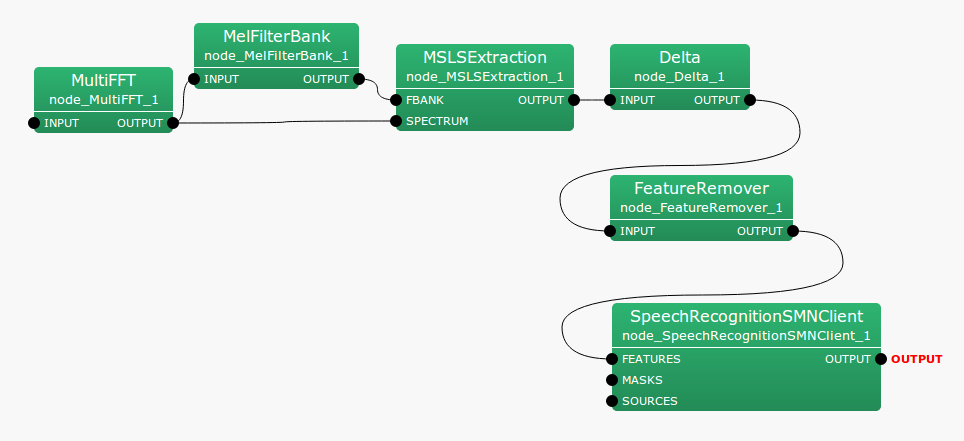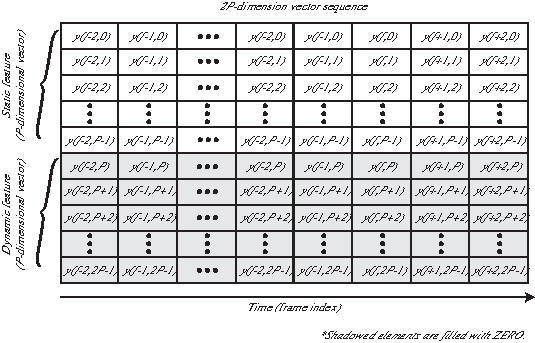## 6.4.5 MSLSExtraction

### 6.4.5.1 Outline of the node

This node acquires mel-scale logarithmic spectra (MSLS), which are a type of acoustic feature, and logarithmic power. It generates acoustic feature vectors consisting of the mel-scale logarithmic spectrum coefficients and logarithmic spectrum power as elements.

### 6.4.5.2 Necessary file

No files are required.

### 6.4.5.3 Usage

When to use

The mel-scale logarithmic spectrum coefficients and logarithmic spectrum power are used as the elements of this node. This node is used to generate acoustic feature vectors. For example, acoustic feature vectors are input to the speech recognition node to identify phonemes and speakers.

Typical connectionFigure 6.83: Typical connection example of MSLSExtraction

### 6.4.5.4 Input-output and property of the node

Table 6.76: Parameter list of MSLSExtraction
 Parameter name Type Default value Unit Description FBANK_COUNT 13 The number of filter banks for input spectrum. The implementation is optimized to the value 13. NORMALIZATION_MODE CEPSTRAL Feature normalization method USE_POWER false Select whether or not to include logarithmic power in features

Input

FBANK

: Map<int, ObjectRef>  type. A pair of the sound source ID and the vector consisting of sound source output energy of the filter bank as Vector<float> type data.

SPECTRUM

: Map<int, ObjectRef>  type. A pair of the sound source ID and the vector consisting of complex spectra as Vector<complex<float> >  type data.

Output

OUTPUT

: Map<int, ObjectRef>  type. A pair of the sound source ID and the vector consisting of MSLS and a logarithmic power term as Vector<float>  type data. This node obtains static features of MSLS, though vectors containing a dynamic feature part are output. The dynamic feature part is set to zero. Figure 6.84 shows the operation.

Parameter

FBANK_COUNT

: int  type. The number of filter banks for the input spectrum. Its range is positive integer. The frequency band 1 bank narrows as the value is raised and acoustic features of high frequency resolution are obtained. Typical set values are from 13 to 24. The current implementation is optimized for the value set at 13. We strongly recommend you use the defalt value. Acoustic features are expressed more finely by setting greater FBANK_COUNT. Precise expression is not necessarily optimal for speech recognition and it depends on the acoustic environment of the utterances.

NORMALIZATION_MODE

: string  type. The user can designate CEPSTRAL or SPECTRAL. The user selects whether or not to perform normalization in the cepstrum domain / spectrum domain.

USE_POWER

: When selecting true, the logarithmic power term is added to acoustic features. When selecting false, it is omitted. It is rare to use the power term for acoustic features though it is assumed that delta logarithmic power is effective for speech recognition. When true is selected, delta logarithmic power is calculated in the posterior half and its result is used as acoustic features.

### 6.4.5.5 Details of the node

This node acquires mel-scale garithmic spectra (MSLS), which are one of the acoustic features, and logarithmic power. It generates acoustic features consisting of the mel-scale garithmic spectrum coefficients and log spectrum power as elements. Output logarithmic energy of each filter bank is input to FBANK input terminal of this node. The calculation method of the MSLS to be output differs depending on the normalization method the user designates. The following are the calculation methods for the output vectors of this node for each normalization method.

CEPSTRAL

: The input to the FBANK terminal is expressed as:

 $\displaystyle \boldsymbol {x}$ $\displaystyle =$ $\displaystyle [ x(0),x(1), \cdots , x(P-1)]^ T$ (142)

Here, $P$ indicates FBANK_COUNT in the dimension number of the input feature vector. The vector output is a $P+1$ dimensional vector and consists of the MSLS coefficient and power term. The dimensions from the first to the $P$ are for MSLS and the dimension $P+1$ is for the power term. The output vector of this node is expressed as;

 $\displaystyle \boldsymbol {y}$ $\displaystyle =$ $\displaystyle [y(0),y(1),\dots ,y(P-1), E]^ T$ (143) $\displaystyle y(p)$ $\displaystyle =$ $\displaystyle \displaystyle \frac{1}{P} \sum _{q=0}^{P-1} \Bigl \{ L(q) \cdot \sum _{r=0}^{P-1} \Bigl \{ \log (x(r)) \cos \Bigl ( \frac{ \pi q (r+0.5)}{P} \Bigr ) \Bigr \} \cos \Bigl ( \frac{\pi q (p+0.5)}{P} \Bigr ) \Bigr \}$ (144)

Here, the liftering coefficient is expressed as;

 $\displaystyle L(p)$ $\displaystyle =$ $\displaystyle \left\{ \begin{array}{ll} 1.0, & (p=0, \dots ,P-1), \\ 0.0, & (p=P, \dots ,2P-1), \\ \end{array} \right.$ (145)

Here, $Q=22$.

SPECTRAL

: The input to the FBANK part is expressed as:

 $\displaystyle \boldsymbol {x}$ $\displaystyle =$ $\displaystyle [ x(0),x(1), \cdots , x(P-1)]^ T$ (146)

Here, $P$ indicates FBANK_COUNT in the dimension number of the input feature vector. The vector output is a $P+1$ dimensional vector and consists of the MSLS coefficient and power term. The first to the $P$th dimensions are for mel-cepstrum coefficients and the dimension $P+1$ is for the power term. The output vector of this node is expressed as:

 $\displaystyle \boldsymbol {y}$ $\displaystyle =$ $\displaystyle [y(0),y(1),\dots ,y(P-1), E]^ T$ (147) $\displaystyle y(p)$ $\displaystyle =$ $\displaystyle \left\{ \begin{array}{ll} ( \log (x(p))- \mu )- 0.9 ( \log (x(p-1))- \mu ), & if~ ~ p=1,\dots ,P-1 \\ \log (x(p), & if ~ ~ p=0, \\ \end{array} \right.$ (148) $\displaystyle \mu$ $\displaystyle =$ $\displaystyle \frac{1}{P} \sum _{q=0}^{P-1} \log (x(q)),$ (149)

Mean subtraction of the frequency direction and peak enhancement processing are applied.

For the logarithmic power term, the input to the SPECTRUM terminal is expressed as:

 $\displaystyle \boldsymbol {s}$ $\displaystyle =$ $\displaystyle [ s(0),s(1), \dots , s(N-1)]^ T$ (150)

Here, $N$ is determined by the size of Map  connected to the SPECTRUM terminal. For Map , assuming that the spectral representation from 0 to $\pi$ is stored in $B$ bins, $N = 2(B-1)$. Now, the power term is expressed as:

 $\displaystyle p$ $\displaystyle =$ $\displaystyle \log \Bigl ( \frac{1}{N} \sum _{n=0}^{N-1} s(n) \Bigr )$ (151)Figure 6.84: Output parameter of MSLSExtraction